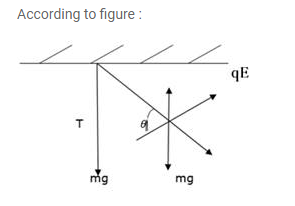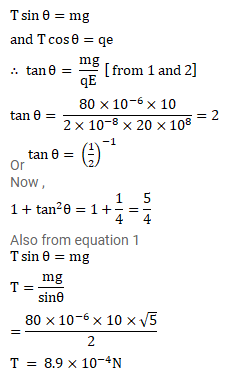# A pendulum bob of mass 80mg and carrying a charge ofQuestion:

A pendulum bob of mass $80 \mathrm{mg}$ and carrying a charge of $2^{\times 10^{-8}} \mathrm{C}$ is at rest in a uniform, horizontal electric field of $20 \mathrm{kV} / \mathrm{m}$. Find the tension in the thread.

Solution: